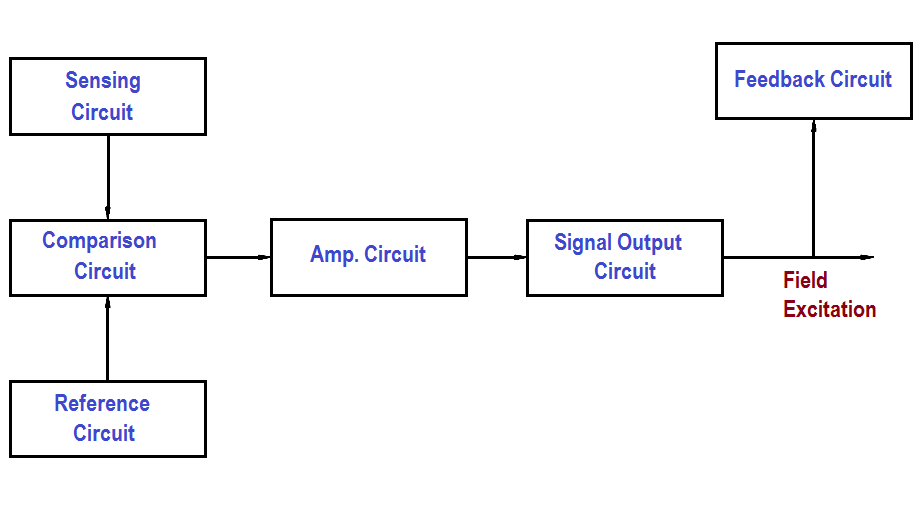# AC Generator Voltage Regulators

Because the voltage from an AC generator varies as the output load and power factor change, a voltage regulator circuit is necessary to permit continuity of the desired output voltage.

#### Purpose

The purpose of a voltage regulator is to maintain the output voltage of a generator at a desired value. As load on an AC generator changes, the voltage will also tend to change. The main reason for this change in voltage is the change in the voltage drop across the armature winding caused by a change in load current. In an AC generator, there is an IR drop and an IXL drop caused by the AC current flowing through the resistance and inductance of the windings.

The IR drop is dependent on the amount of the load change only. The IXL drop is dependent on not only the load change, but also the power factor of the circuit. Therefore, the output voltage of an AC generator varies with both changes in load (i.e., current) and changes in power factor. Because of changes in voltage, due to changes in load and changes in power factor, AC generators require some auxiliary means of regulating output voltage.

#### Block Diagram Description

The below Figure shows a typical block diagram of an AC generator voltage regulator. This regulator consists of six basic circuits that together regulate the output voltage of an AC generator from no-load to full-load.Figure : Voltage Regulator Block Diagram

#### Sensing Circuit

The sensing circuit senses output voltage of the AC generator. As the generator is loaded or unloaded, the output voltage changes, and the sensing circuit provides a signal of these voltage changes. This signal is proportional to output voltage and is sent to the comparison circuit.

#### Reference Circuit

The reference circuit maintains a constant output for reference. This reference is the desired voltage output of the AC generator.

#### Comparison Circuit

The comparison circuit electrically compares the reference voltage to the sensed voltage and provides an error signal. This error signal represents an increase or decrease in output voltage. The signal is sent to the amplification circuit.

#### Amplification Circuit

The amplification circuit, which can be a magnetic amplifier or transistor amplifier, takes the signal from the comparison circuit and amplifies the milliamp input to an amp output, which is then sent to the signal output, or field, circuit.

#### Signal Output Circuit

The signal output circuit, which controls field excitation of the AC generator, increases or decreases field excitation to either raise or lower the AC output voltage.

#### Feedback Circuit

The feedback circuit takes some of the output of the signal output circuit and feeds it back to the amplification circuit. It does this to prevent overshooting or undershooting of the desired voltage by slowing down the circuit response.

#### Changing Output Voltage

Let us consider an increase in generator load and, thereby, a drop in output voltage. First, the sensing circuit senses the decrease in output voltage as compared to the reference and lowers its input to the comparison circuit. Since the reference circuit is always a constant, the comparison circuit will develop an error signal due to the difference between the sensed voltage and the reference voltage.

The error signal developed will be of a positive value with the magnitude of the signal dependent on the difference between the sensed voltage and the reference voltage. This output from the comparison circuit will then be amplified by the amplifier circuit and sent to the signal output circuit. The signal output circuit then increases field excitation to the AC generator. This increase in field excitation causes generated voltage to increase to the desired output.

If the load on the generator were decreased, the voltage output of the machine would rise. The actions of the voltage regulator would then be the opposite of that for a lowering output voltage. In this case, the comparison circuit will develop a negative error signal whose magnitude is again dependent on the difference between the sensed voltage and the reference voltage. As a result, the signal output circuit will decrease field excitation to the AC generator, causing the generated voltage to decrease to the desired output.